IP address is a numeric identifier that is assigned to a device for communicate with other devices on a network. IP address consists of four decimal numbers which are separated by dots or decimal points. Hence, we call dotted-decimal notation. For example, 192.168.0.1 is an IP address written in dotted-decimal notation, but the binary version is 11000000 10101000 00000000 00000001 (IP address is stored as binary numbers). You will see how to convert between binary and decimal numbers later.Each decimal numbers of an IP address represents 8-bit (or 1 byte), and is therefore called an octet. Hence, an IP address represents 32-bit (or 4 bytes). The range of each octet is between 0 and 255 inclusively.

Formats/Octets First Second Third Fourth
Binary 11000000 10101000 00000000 00000001
Decimal 192 168 0 1

## Classes of Networks

For better understanding in IP addressing, let’s see a sample network diagram below.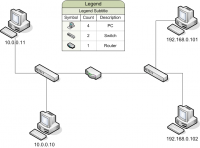On this diagram, there are 2 networks: 10.0.0.0 and 192.168.0.0 which is separated by a router. At this time, you may want to know how can I know that 192.168.0.101 and 192.168.0.102 are on the same network. Or if I want to add new PC on 10.0.0.0 network, what are valid IP addresses that I can choose? The answer is about classes of networks.

An IP address can be divided in 2 parts, network and host. RFC 790 defines IP protocol, including network classes which are Class A, Class B, and Class C. TCP/IP defines Class D and Class E as well. See the table below for details on each class.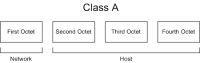Class A, network part has 1 byte (8 bits) and 3 bytes (24 bytes) for host part. *Total number of this class of network: 2 ^ 7 – 2 Valid network numbers: 1.0.0.0 to 126.0.0.0 **Number of hosts per network: 2 ^ 24 – 2 Valid host addresses: 1.0.0.1 to 126.255.255.254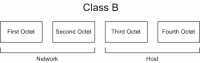Class B, network part has 2 bytes (16 bits) and 2 bytes (16 bits) for host part. *Total number of this class of network: 2 ^ 14 – 2 Valid Network numbers: 128.1.0.0 to 191.254.0.0 **Number of hosts per network: 2 ^ 16 – 2 Valid host addresses: 128.1.0.1 to 191.255.255.254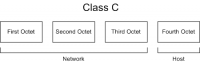Class C, network part has 3 bytes (24 bits) and 1 byte (8 bits) for host part. *Total number of this class of network: 2 ^ 21 – 2 Valid Network numbers: 192.0.1.0 to 223.255.254.0 **Number of hosts per network: 2 ^ 8 – 2 Valid host addresses: 192.0.1.1 to 223.255.254.254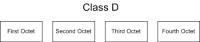Class D is reserved for multicast address and used for communicate between router or gateway. Valid IP addresses: 224.0.0.0 to 239.255.255.255.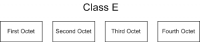Class E is reserved for experiment, development purpose, or future use. Valid IP addresses: 240.0.0.0 to 254.255.255.254.

Note:
*The valid network numbers shows actual network numbers. There are several reserved cases. For example, network 0.0.0.0, originally defined for use as a broadcast address, and 127.0.0.0, reserved for loopback address. Network 128.0.0.0, 191.255.0.0, 192.0.0.0 and 223.255.255.0 also are reserved.

Network mask or NetMask is a 32-bit binary number, usually written in dotted-decimal format. Network mask defines the size of the host part of an IP address, representing the host part of the IP address with binary 0s in the mask. For example, class A mask has its last 24 bits as binary 0, which means that the last three octets of the mask are 0s. You can see the default masks on the table below.

Class Size of network part, in bits Size of host part, in bits Default mask
A 8 24 255.0.0.0
B 16 16 255.255.0.0
C 24 8 255.255.255.0

Network address or network number is a number that uses dotted-decimal notation like IP addresses, but the number itself represents all hosts in a single Class A, B, or C IP network. For example, given an IP address 192.168.0.1 with network mask 255.255.255.0, the network address will be 192.168.0.0.

To calculate network address, we use logical AND operation between one IP address (any) in the network and its network mask. For example, an IP address 192.168.0.1 with network mask 255.255.255.0.

1. Convert the IP address 192.168.0.1 to binary format, we get 11000000 10101000 00000000 00000001.
2. Convert its network mask 255.255.255.0 to binary format, we get 11111111 11111111 11111111 00000000
3. Do logical AND operation on two binary numbers, we get 11000000 10101000 00000000 00000000.
Note: A logical AND operation compares 2 bits. In each pair, the result is 1 if the first bit is 1 and the second bit is 1. Otherwise, the result is 0.
4. Convert the result back to decimal, we get 192.168.0.0.

You can see the example on the figure below: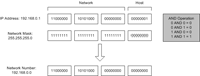Broadcast Address or Direct Broadcast Address is an IP address which refers to all nodes on a network or subnet instead of a single node. For example, a network address 192.168.0.0 with network mask 255.255.255.0, the broadcast address is 192.168.0.255 which refers to 192.168.0.1 to 192.168.0.254. If you send a packet to 192.168.0.255, all nodes on this network (192.168.0.1 to 192.168.0.254) will get the packet.

1. Convert network mask 255.255.255.0 to binary format, we get 11111111 11111111 11111111 00000000.
2. Do bit inversion on the network mask, we get 00000000 00000000 00000000 11111111.
3. Convert network address 192.168.0.0 to binary format, we get 11000000 10101000 00000000 00000000.
4. Do logical XOR operation on two binary numbers, we get 11000000 10101000 00000000 11111111.
Note: A logical XOR (exclusive or) is a type of logical disjunction on two operands that results in a value of true if exactly one of the operands has a value of true. A simple way to state this is “one or the other but not both.”
5. Convert the result back to decimal, we get 192.168.0.255.

You can see the example on the figure below: